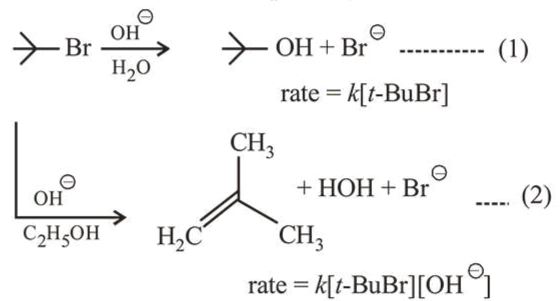# Consider the reaction sequence given below :

Question:

Consider the reaction sequence given below :Which of the following statements is true?

1. (1) Changing the base from $\mathrm{OH}^{\ominus}$ to $^{\ominus} \mathrm{OR}$ will have no effect on reaction (2).

2. Changing the concentration of base will have no effect on reaction (1).

3. Doubling the concentration of base will double the rate of both the reactions.

4. Changing the concentration of base will have no effect on reaction (2).

Correct Option: , 2

Solution:

First reaction is $\mathrm{S}_{\mathrm{N}} 1$ in which rate does not depend on conc. of nucleophile but depends on reactant conc. Second reaction is E2 reaction in which rate depends on conc. of base as well as reactant conc.

Therefore, changing the concentration of base will have no effect on rate of reaction (1).